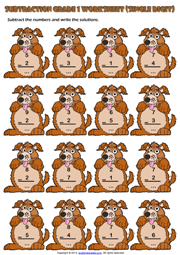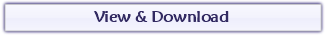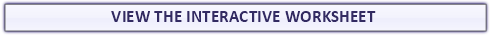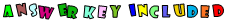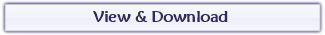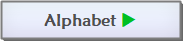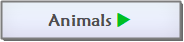Found a mistake?
!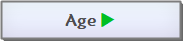## Single Digit Subtraction Maths Exercise WorksheetA fun single digit subtraction exercise maths worksheet for grade 1 (first grade) students and kids with rabbit and canvas theme. Write the solutions and colour the rabbit. (Vertical Subtraction Worksheet for kids)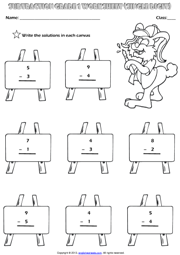Count the fruits, fill in the blanks with their numbers and find the solutions. A colorful subtraction exercise maths worksheet with fruit theme for grade 1 (first grade) students and kids. (Horizontal Subtraction Worksheet for first grade students with enjoyable pictures)

## Count and Subtract Picture Maths Exercise Worksheet## First Grade Single Digit Subtraction Math Exercise Worksheet

A simple single digit subtraction exercise maths worksheet for grade 1 (first grade) students and kids with rainbow theme. Subtract the numbers and write the solutions. (Horizontal Subtraction Worksheet for first grade students)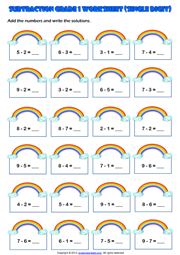A practical subtraction exercise maths handout for grade 1 (first grade) students and kids with dogs theme. Subtract the numbers and write the solutions. (Vertical Subtraction Worksheet for first grade students)

## First Grade Single Digit Subtraction Maths Exercise Handout